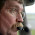Saturday, April 19, 2014

Si570 Programming

Messing around with the Si570 chip that is used in the Minima transceiver designed by Farhan Akhtar.  This is my lash-up:

After a bit of tweaking, I have the oscillator working.  It is no picnic trying to interpret the Si570 datasheet however.

Interestingly enough most of the code implementations I have looked at for the Arduino make a couple of errors in interpretation of the datasheet.  The Si570 is not really fun to program, but it has a number of benefits going for it.

My particular chip is a 10-160 MHz CMOS model.  When I first power it up, it comes up at 56.320 MHz.  The datasheet is not explicit on this, but supposedly the individual parts are "tuned" at the factory to their default frequency by adjusting the various parameters that are used to set the frequency.  However, the datasheet does not provide very flattering stability numbers and nowhere does it provide a statement of how closely it is calibrated in the factory.

So, in the absence of highly accurate frequency measurement capabilities you are faced with having to calculate the internal crystal frequency based on the default output frequency (from the datasheet) after reading the frequency setting parameters from the chip itself.

The Si570 requires mathematical calculations with 38 bits of precision in order to accurately set it's frequency.  Most Arduino implementations rely on double precision floating point math functionality.  However, the Arduino implementation of double precision is in fact identical to its single precision floating point.  Namely double = float data types silently behind the scenes.  So, most of these implementations give up on the number of digits of precision required to control the Si570 even down to the 1 Hz level.  Theoretically you should be able to set the frequency to within .42 Hz, but the unfortunate floating point double precision implementation on Arduino prevents even 1 Hz resolution.

The Si570 stores a reference frequency used in the output frequency calculation as a 38 bit values where 10 bits are use as a decimal multiplier.  The remaining 28 bits are a fractional multiplier stored as an integer value scaled by 2^28.

The internal DCO is constrained to 4.85 to 5.67 GHz determined by the internal crystal frequency multiplied by a reference frequency.  According to the settings in my chip, I calculate my crystal frequency to be 114.252365 MHz.  Secondarily, frequency excursions of the DCO can be done in a phase continuous manner if the change in frequency is no more than +/- 3500 PPM.  Many implementations however are calculating this based on the output frequency rather than the DCO frequency in error.  Frequency excursions larger than this require the DCO to be stopped and restarted in a non-phase continuous manner that can take upwards of 10 ms to complete.  Circuitry sensitive to runt pulses may require resetting after this operation.

The output frequency is the DCO frequency divided by the product of two divisors.  There are additional restrictions on these two divisors.

More to follow...

1.1.